Thursday, June 4, 2020
Home > Latest Announcement > Maths Tricky Questions With Solutions Set 1

# Maths Tricky Questions With Solutions Set 1# Maths Tricky Questions with Solutions Set 1

In this post, the following Maths Tricky Questions with Solutions Set 1 is given.

## Maths Tricky Questions with Solution Set 1 : AMBQID 1

An equilateral triangle is surrounded by three squares as shown in the figure. Find the value of x?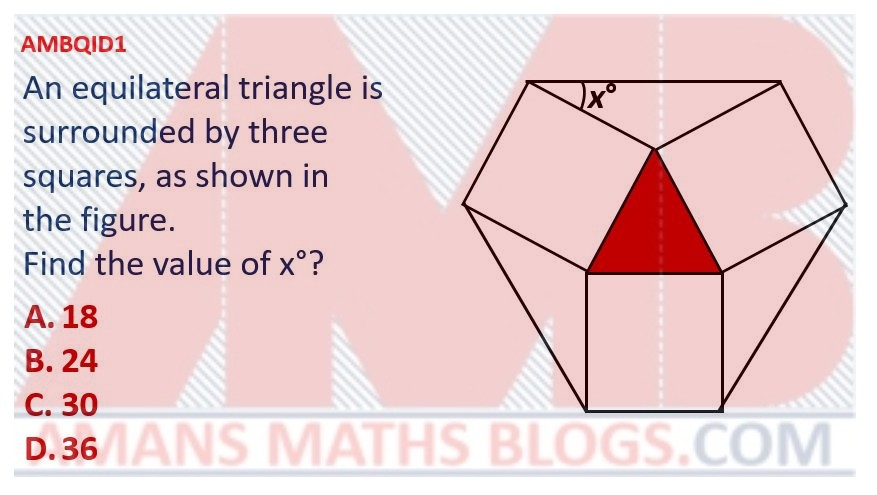Options:

A. 18

B. 24

C. 30

D. 36

Solution:

## Maths Tricky Questions with Solution Set 1 : AMBQID 2

If x and y are whole numbers such that x2 + 101x = y2, then find the maximum value of y.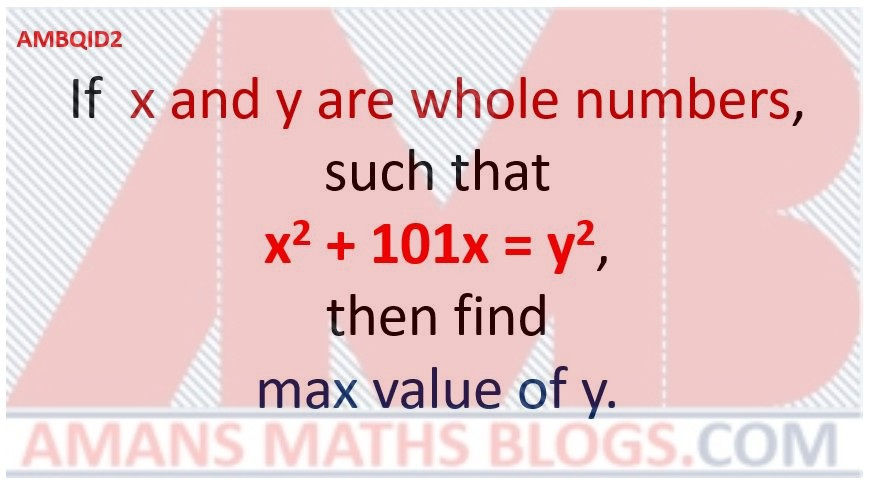Options:

A.

B.

C.

D.

Solution:

## Maths Tricky Questions with Solution Set 1 : AMBQID 3

Find the value of.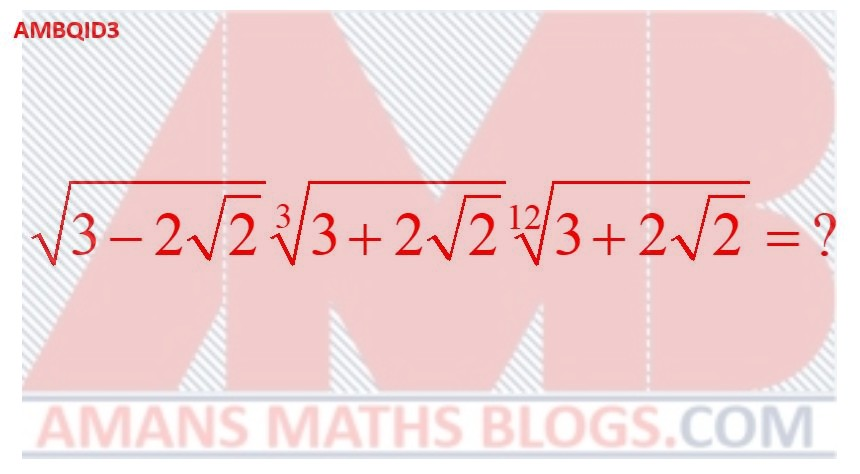Options:

A.

B.

C.

D.

Solution:

## Maths Tricky Questions with Solution Set 1 : AMBQID 4

In the given figure, all the quadrilateral are squares. Find the ratio of ar(Red Square) : ar(Green Square)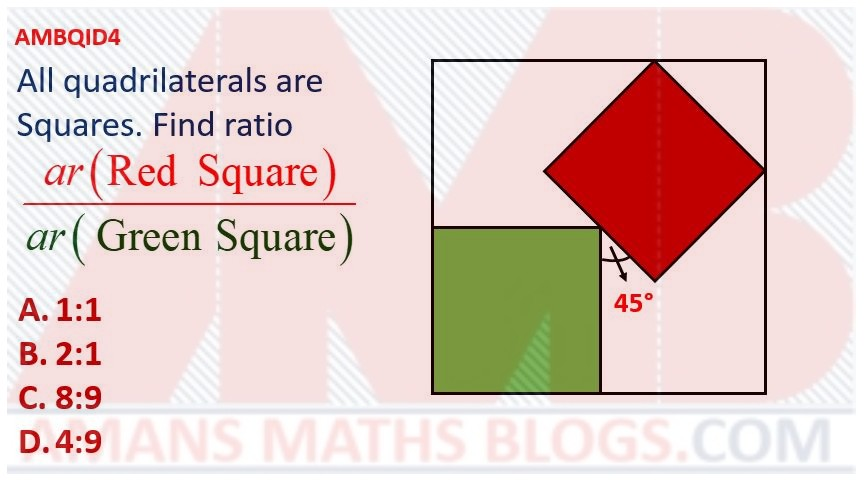Options:

A. 1 : 1

B. 2 : 1

C. 8 : 9

D. 4 : 9

Solution:

## Maths Tricky Questions with Solution Set 1 : AMBQID 5

In circle, C is the center. If ar(PQR) = 2ar(PSR), then find the angle PRS.Options:

A. 18

B. 22.5

C. 15

D. 30

Solution:

## Maths Tricky Questions with Solution Set 1 : AMBQID 6

In a square ABCD, P, Q, R, S are id-points of sides as shown. If ar(ABCD) = 1 sq. unit, then find shaded green area.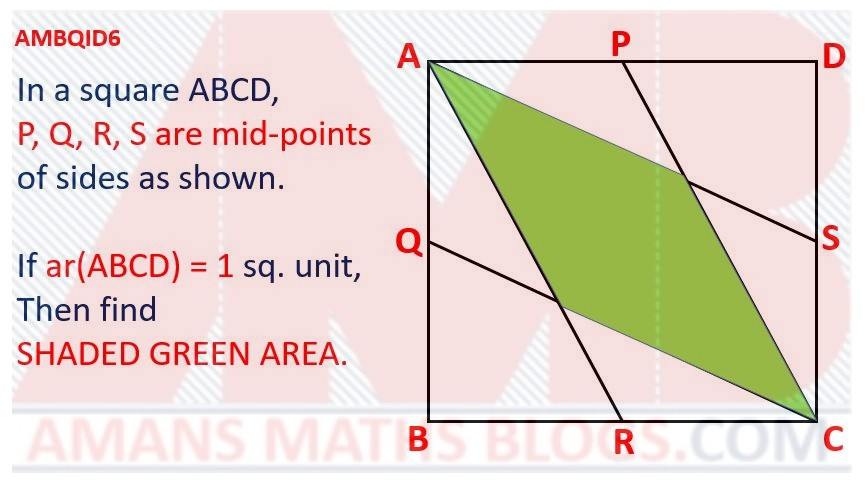Options:

A.

B.

C.

D.

Solution:

## Maths Tricky Questions with Solution Set 1 : AMBQID 7

In the figure, the angle ACB = 70 degree and the angle CAD = 30 degree. If BC = AD, then find the angle ABC.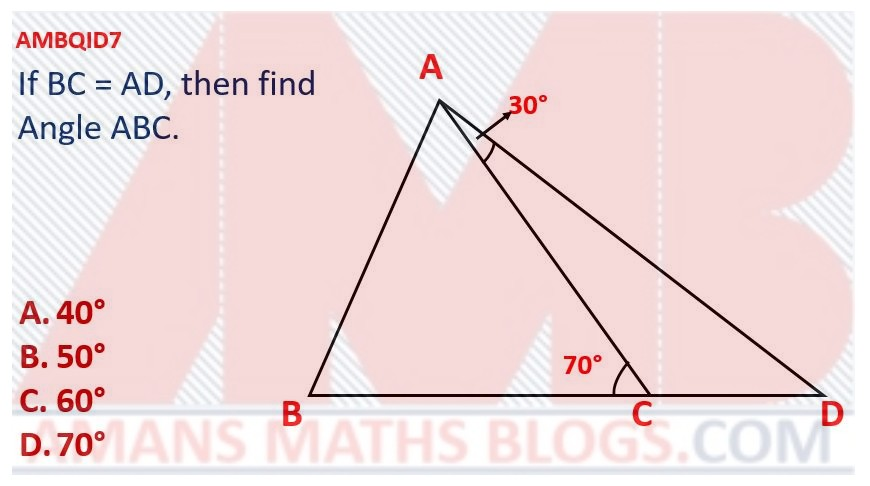Options:

A. 40 degree

B. 50 degree

C. 60 degree

D. 70 degree

Solution:

## Maths Tricky Questions with Solution Set 1 : AMBQID 8

In the figure, if the area inside the circle but outside the triangle is equal to the area inside the triangle but outside the circle, then find the radius of the circle.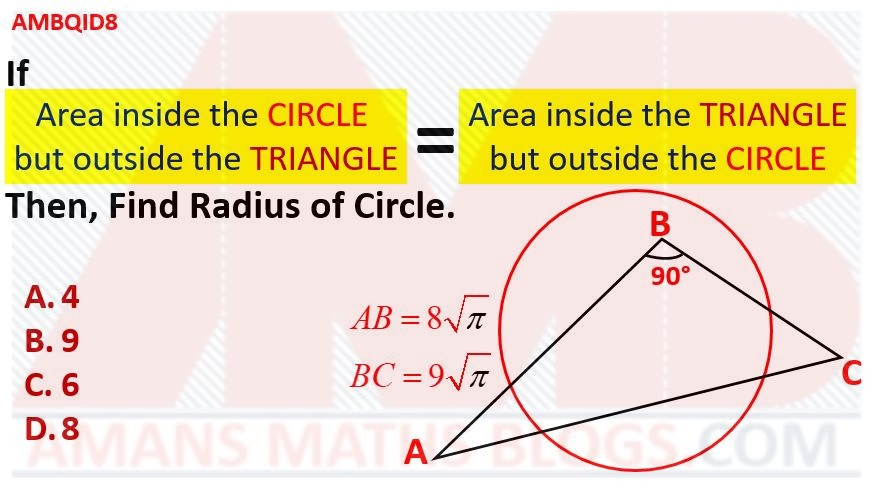Options:

A. 4

B. 9

C. 6

D. 8

Solution:

## Maths Tricky Questions with Solution Set 1 : AMBQID 9

In triangle PQR, I (Incenter), O (Orthocenter), C (Circumcenter), Q and R (Vertices) are lie on a circle, then find the angle P.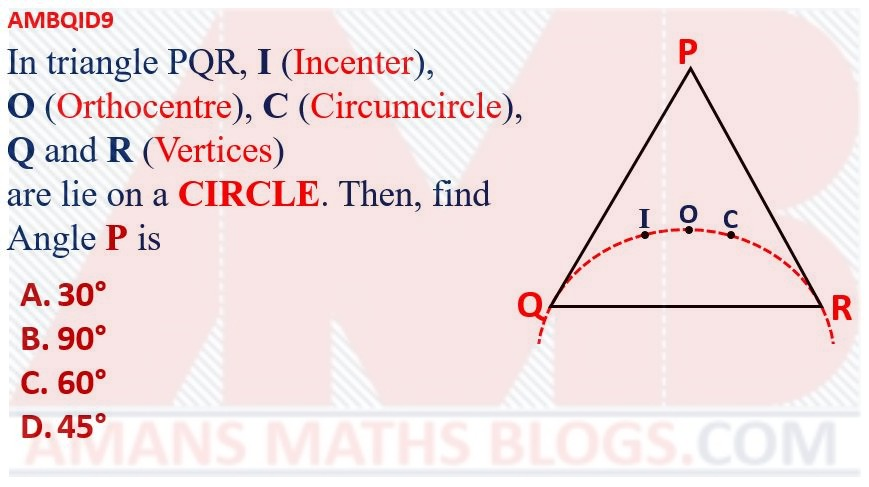Options:

A. 30 degree

B. 90 degree

C. 60 degree

D. 45 degree

Solution:

## Maths Tricky Questions with Solution Set 1 : AMBQID 10

If 4x = 9 and 9y = 256, then find the value of xy.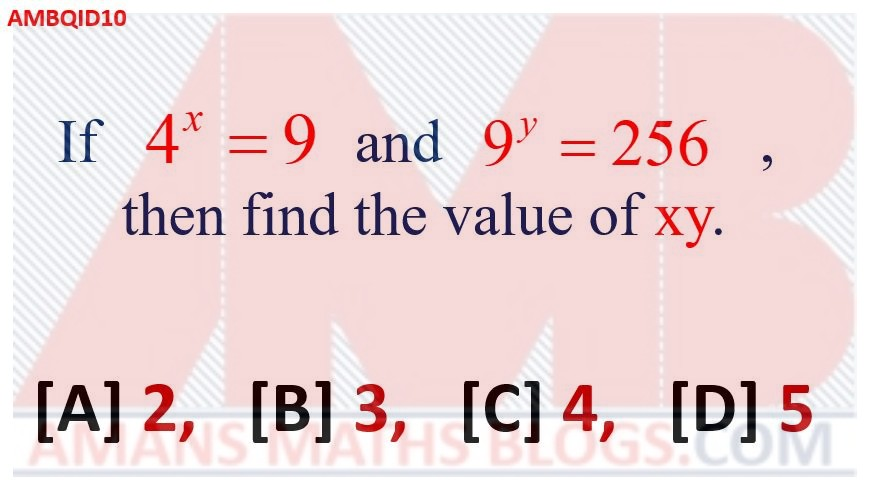Options:

A. 2

B. 3

C. 4

D. 5

Solution:

## Maths Tricky Questions with Solutions Set 1 : AMBQID 11

A square is inside an equilateral triangle. Find the value of (x + y).Options:

A. 100

B. 120

C. 150

D. 165

Solution:

## Maths Tricky Questions with Solution Set 1 : AMBQID 12

If two positive integers x and y are the length and breadth of a rectangle respectively such that the perimeter of rectangle is equal to the area of rectangle, then find the value of (x – y).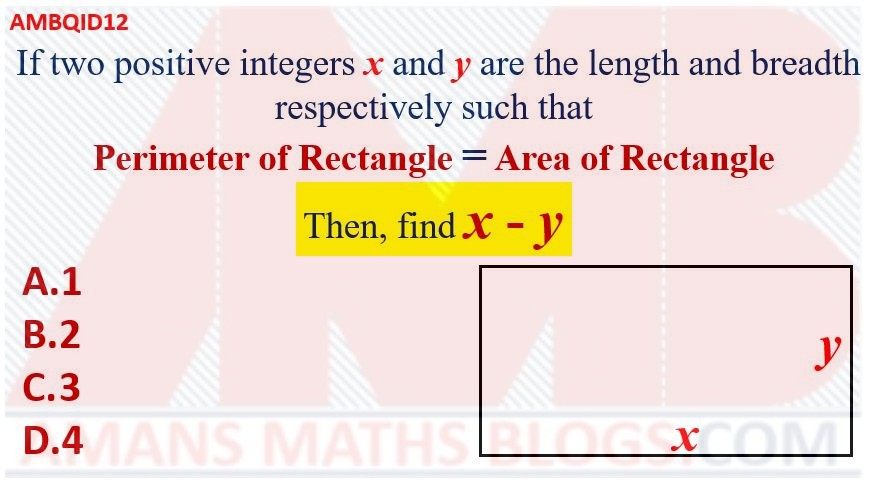Options:

A. 1

B. 2

C. 3

D. 4

Solution:

## Maths Tricky Questions with Solutions Set 1 : AMBQID 13

In the figure, if CD = 32 and the shaded area is k(PI), then find the value of k.Options:

A. 128

B. 132

C. 153

D. 168

Solution:

## Maths Tricky Questions with Solution Set 1 : AMBQID 14

In 10 sided regular polygon, find the value of x.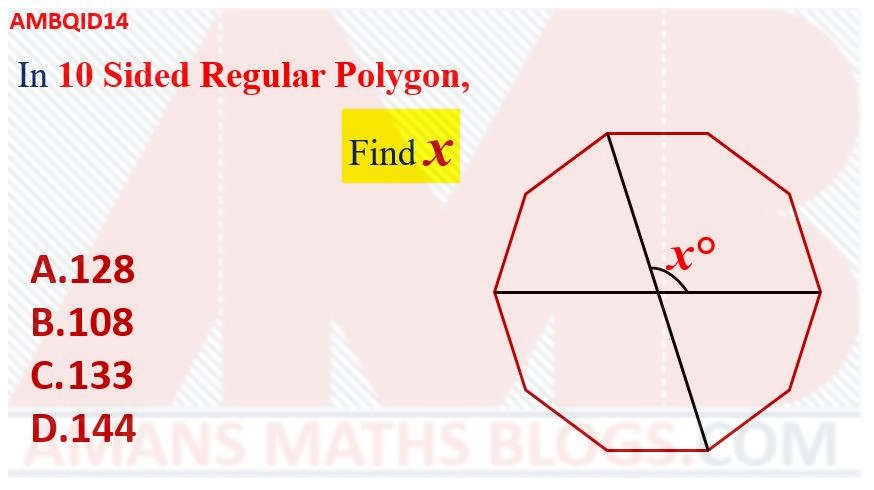Options:

A. 128

B. 108

C. 133

D. 144

Solution:

## Maths Tricky Questions with Solutions Set 1 : AMBQID 15

If ar(ABCD) = 243, then find the value of R.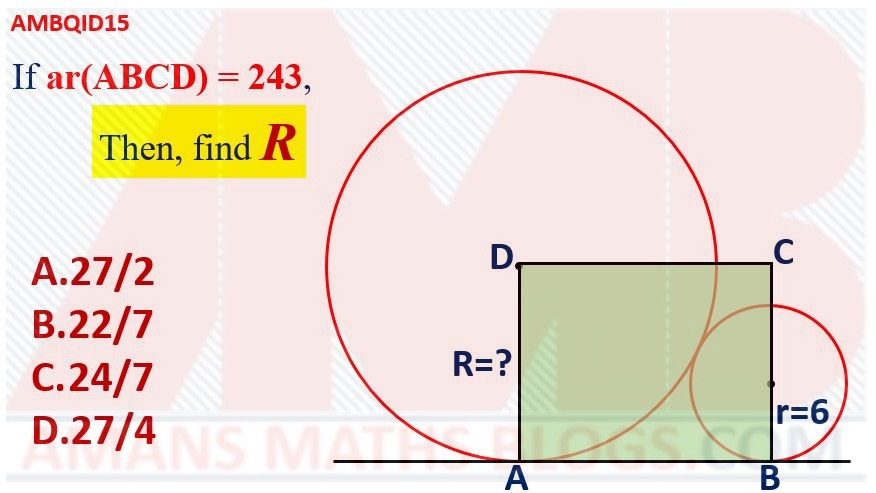Options:

A. 27/2

B. 22/7

D. 27/4

Solution:

## Maths Tricky Questions with Solution Set 1 : AMBQID 16

Find the number of integr solutions of the equation 3x + 2/x = -7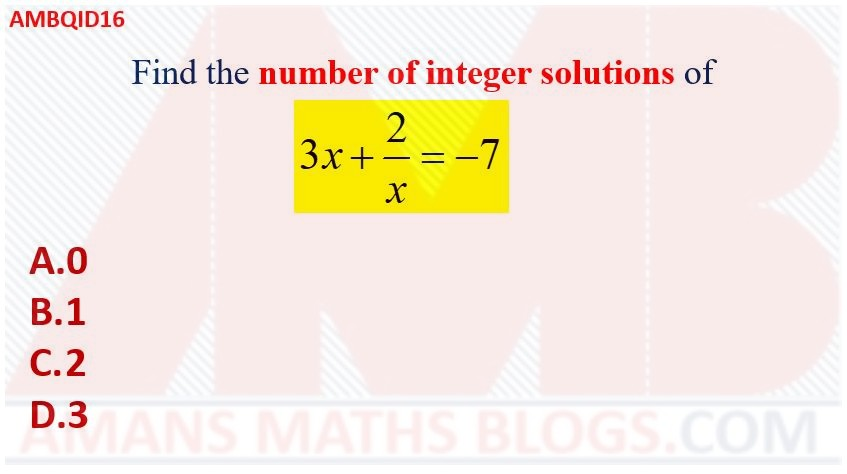Options:

A. 0

B. 1

C. 2

D. 3

Solution:

## Maths Tricky Questions with Solutions Set 1 : AMBQID 17

An equilateral triangle is inside a regular octagon. Then, find the value of x.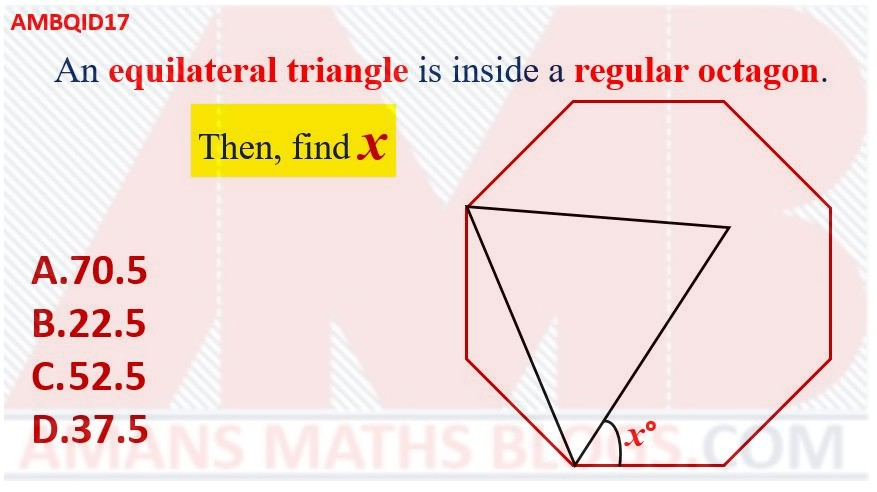Options:

A. 70.5

B. 22.5

C. 52.5

D. 37.5

Solution:

## Maths Tricky Questions with Solution Set 1 : AMBQID 18

In the given figure, the red shaded area is equal to the blue shaded area. If the side of the square is, then find the radius of the circle.Options:

A. 7

B. 2

C. 5

D. 4

Solution:

## Maths Tricky Questions with Solutions Set 1 : AMBQID 19

If the red shaded area = 18 cm2, then find the value of xOptions:

A. -8

B. 3

C. 2

D. -6

Solution:

## Maths Tricky Questions with Solution Set 1 : AMBQID 20

If red shaded area = green shaded area = blue shaded area, then find the value of AB/BCOptions:

A. 1

B.C.D. 1/Solution:

## Maths Tricky Questions with Solution Set 1 : AMBQID 21

If (a2 + 1)(b2 + 1) + 16 = 8(a + b), then find the value of (a3 + b3).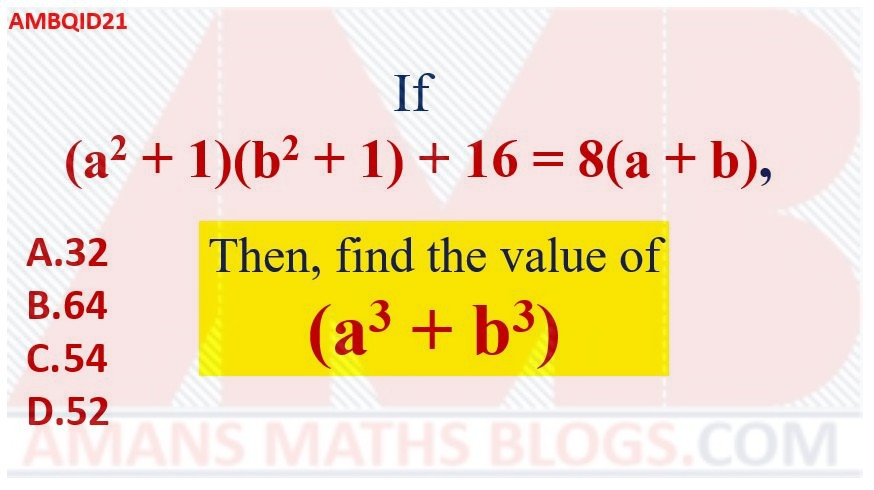Options:

A. 1

B.C.D. 1/Solution:

## Maths Tricky Questions with Solutions Set 1 : AMBQID 22

In the given figure, find the green shaded area.Options:

A. 3Pi

B. 6Pi

C. 5Pi

D. 4Pi

Solution:

## Maths Tricky Questions with Solution Set 1 : AMBQID 23

If AB = 12 Units, then find the yellow shaded area.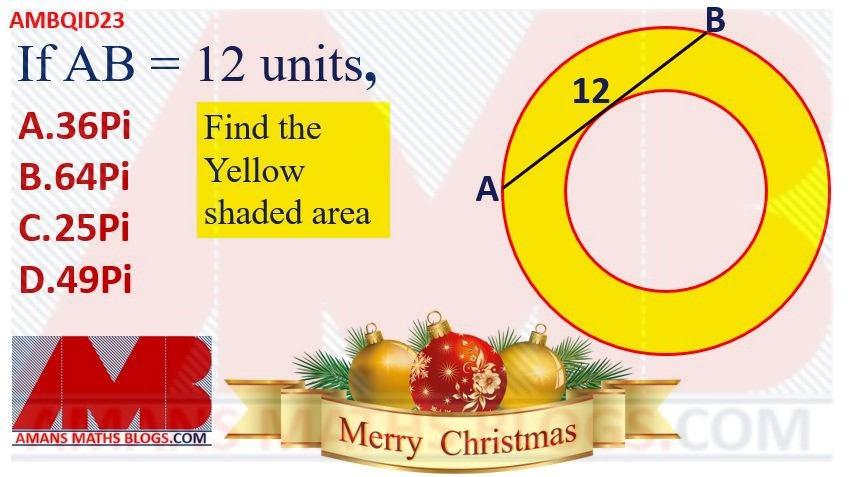Options:

A. 36Pi

B. 64Pi

C. 25Pi

D. 49Pi

Solution:

## Maths Tricky Questions with Solutions Set 1 : AMBQID 24

If a is the one of the roots of the equation x2 + 2x + 3 = 0, then find the value of (a5 + 3a4 + 3a3 – a2)/(a2 + 3)Options:

A. 3

B. 4

C. 5

D. 2

Solution:

## Maths Tricky Questions with Solution Set 1 : AMBQID 25

If a, b, c are the positive integers such that a + 11/b + c/4 = 20, then find the maximum (a + b + c).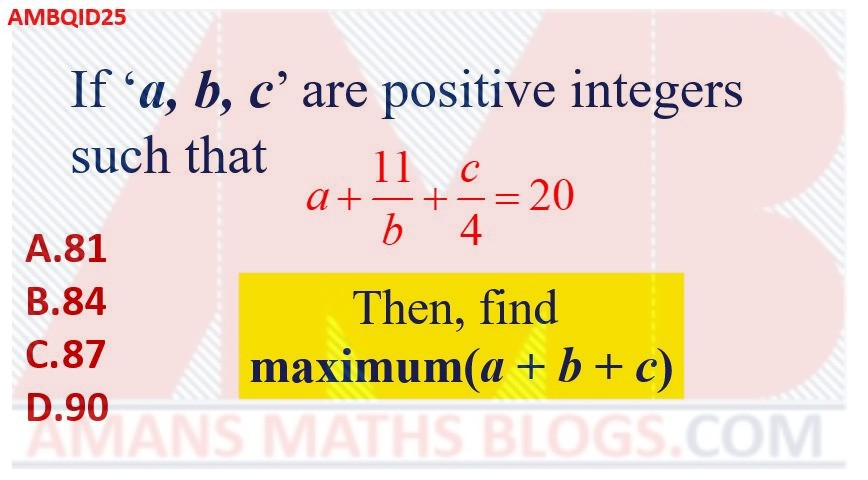Options:

A. 81

B. 84

C. 87

D. 90

Solution:

## Maths Tricky Questions with Solutions Set 1 : AMBQID 26

In the given figure, there is a pattern of four identical rhombuses. Then, find the value of x.Options:

A. 25

B. 65

C. 115

D. 125

Solution:

## Maths Tricky Questions with Solution Set 1 : AMBQID 27

In the triangle ABC, PQ || MN such that AP : PB = 5:3 and AM:MC = 1:4, BQ = 6 and NC = 12. Then find QN.Options:

A. 12

B. 13

C. 14

D. 15

Solution:

## Maths Tricky Questions with Solutions Set 1 : AMBQID 28

In the right triangle ABC, A is the right angle and M is the midpoint of AC. If AP is perpendicular to BM, angle ACB = alpha and angle PAM = beeta, then find the value of tan(alpha)tan(beeta)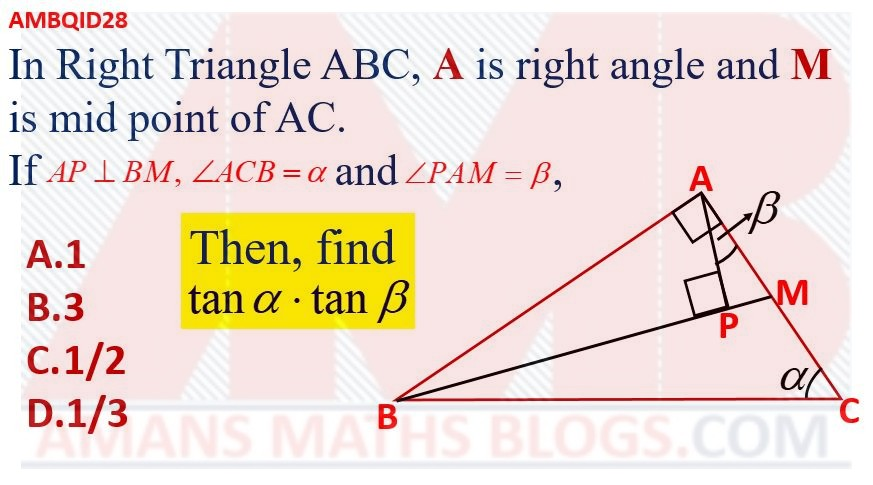Options:

A. 1

B. 3

C. 1/2

D. 1/3

Solution:

## Maths Tricky Questions with Solution Set 1 : AMBQID 29

In the given figure, the triangle ABC is an isosceles triangle with AB = AC and BL is perpendicular to AC. If BL = 4 cm and BC = 5 cm, then find the value of area of the triangle ABC (in cm2).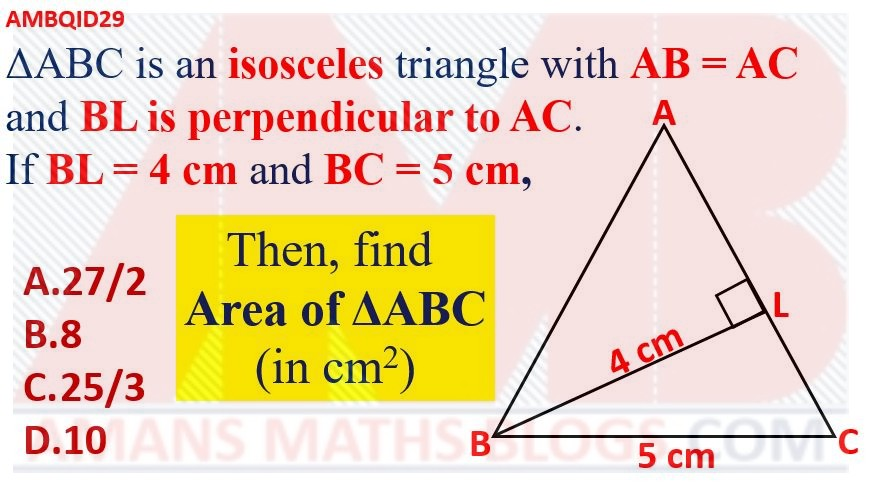Options:

A. 27/2

B. 8

C. 25/3

D. 10

Solution:

## Maths Tricky Questions with Solution Set 1 : AMBQID 30

Find the value of (x2 – y2)2/[(1 – x2)(y2 – 1)] + (1 – x2)2/[(x2 – y2)(y2 – 1)]+(y2 – 1)2/[(x2 – y2)(y2 – 1)]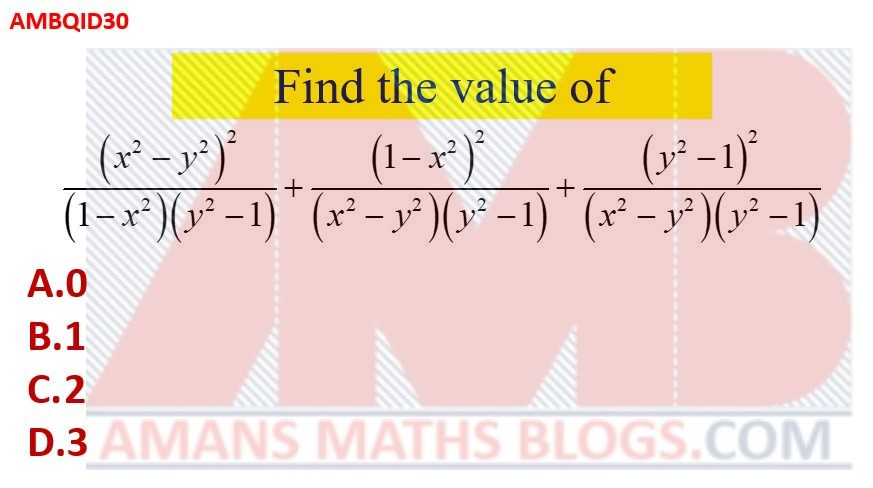Options:

A. 0

B. 1

C. 2

D. 3

Solution:

error: Content is protected !!